Author: Oscar Cronquist Article last updated on April 26, 2021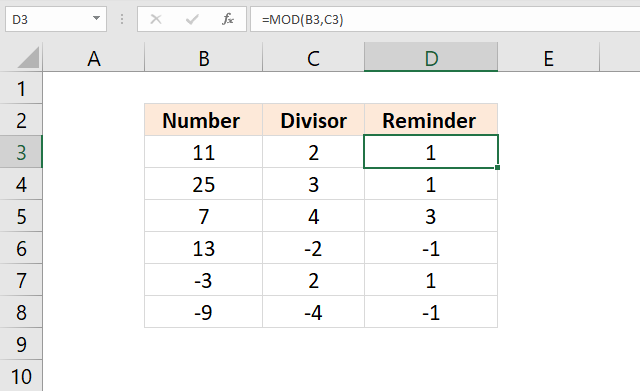The Mod function returns the remainder after a number is divided by a divisor. The Mod function is short for the Modulo operation (wikipedia).

The image above shows that the remainder of 11/2 is 1. 5*2 = 10. 11-10 = 1.

## Excel Function Syntax

MOD(number, divisor)

## Arguments

 number Required. The number for which you want to find the remainder. divisor Required. Divisor is number by which you want to divide the number (first argument).

## 1. What is the remainder after a division?

The remainder is what is left after a division. If you divide 15 with 2 you get 7 and 1 is left over. 2*7 equals 14 and 15 minus 14 equals 1. 1 is the remainder or is left over. MOD(15,2) = 1. Sometimes nothing is left over, like 6 divided by 3 equals 2. The remainder is 0.

MOD(number, divisor) can be expressed like this: number - divisor*INT(number/divisor)

### Example 1,

11 divided by 3 returns 2.

number - divisor*INT(number/divisor)

11-3*INT(11/3)

becomes

11-3*3

becomes

11-9 equals 2.

### Example 2,

Here is a different approach.

14 divided by 5 is 2 and 4/5

Take the remainder (4/5) and multiply it with the divisor (5)

5* (4/5) equals 4. 4 is the answer.

Back to top

### Example 3,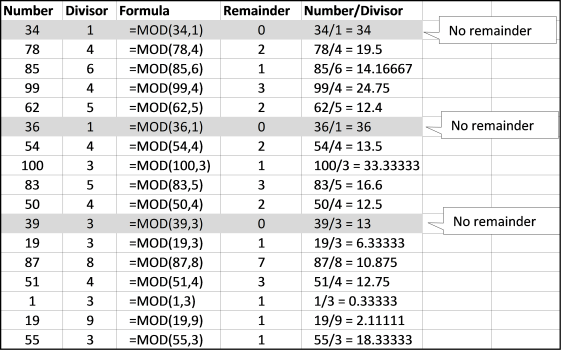The image above demonstrates the result the MOD function returns using different numbers in both arguments number and divisor.

Back to top

## 2. How to calculate a formula for every n-th row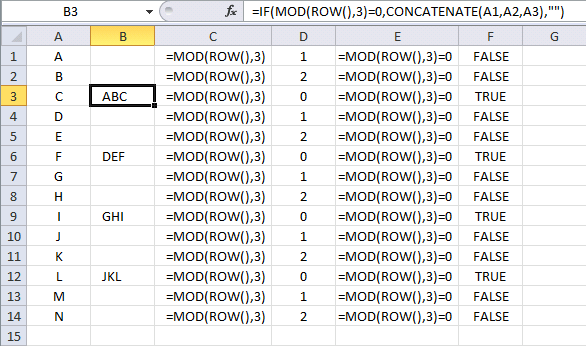The following formula concatenates cells in column A every third row:

=IF(MOD(ROW(), 3)=0, CONCATENATE(A1, A2, A3), "")

Lets begin with ROW() in cell B3. It is dynamic and changes for each row. It returns the current row number, example in cell B3 ROW() returns 3.

Then the MOD function takes 3 and divides it with 3. MOD(ROW(), 3) returns 0.  The remainder is zero in cell B3. You can see this part of the formula in column C and the result in column D.

MOD(ROW(),3)=0 is a logical expression, it checks if the result from the MOD function is equal to 0 (zero). In every third row it is equal and returns TRUE, MOD(ROW(),3)=0 returns TRUE. You can see this part of the formula in column E and the result in column F.

The IF function returns CONCATENATE(A1, A2, A3) if the logical expression is TRUE or a blank if the logical expression is FALSE.

There are relative cell references in the CONCATENATE function and they change in each cell. Don't know much about relative and absolute cell references? Read this: Absolute and relative references in excel

=IF(MOD(ROW(), 3)=0, CONCATENATE(A1, A2, A3), "")

Back to top

## 3. Highlight every n-th rowYou can use the same technique to highlight every second row with conditional formatting. Here is how to apply conditional formatting to a cell range.

Select a cell range. Go to the "Home" tab on the ribbon. Click the Conditional formatting button. Click "New Rule...". Click "Use a formula to determine which cells to format:".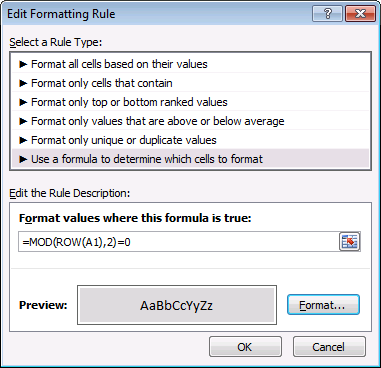Type the formula below. Click the "Format" button. Go to tab "Fill". Pick a color. Click OK twice.

Conditional formatting formula:

=MOD(ROW(A1),2)=0

If you want to highlight every third row change the formula to =MOD(ROW(A1),3)=0.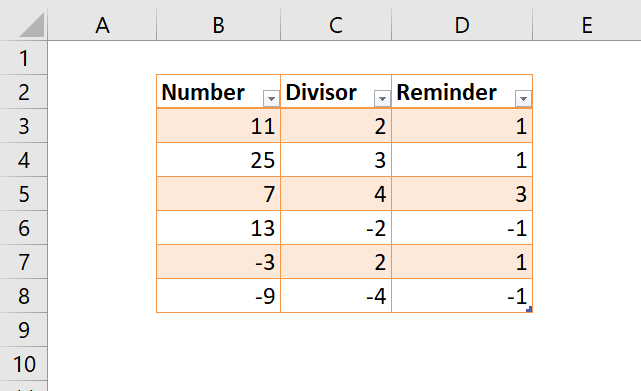Tip! Use Excel Tables to automatically format every other row if you don't want to use formulas and Conditional Formatting. You can easily change the formatting, select any cell in the Excel Table. A new tab appears on the ribbon named "Table Desing".Click on tab "Table Design". Click on any table style to quickly change formatting.

Back to top

## 4. Highlight every other group, example 1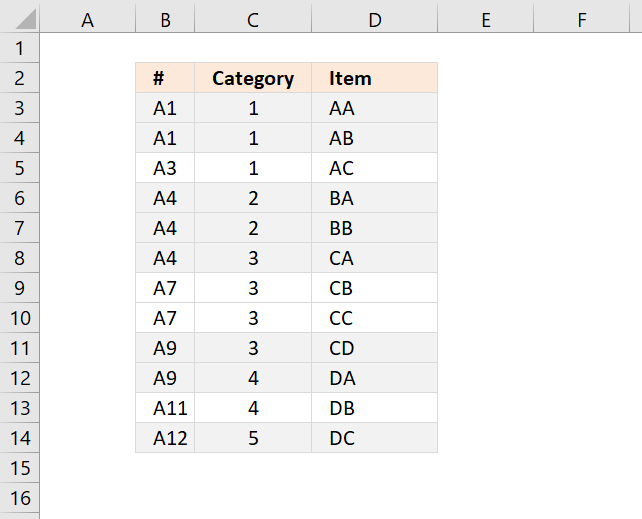This conditional formatting rule highlights every other group based on values in column B. Column B must be sorted.

Conditional formatting formula:

=MOD(SUM(1/COUNTIF(\$B\$2:\$B2,\$B\$2:\$B2)),2)

### Explaining CF formula in cell B3

I recommend that you enter the formula in cell F3 to be able to evaluate the formula using the "Evaluate Formula" tool. The formula returns either TRUE or FALSE and the row is highlighted if it returns TRUE.

To start the "Evaluate Formula" tool go to tab "Formulas" on the ribbon.  Click the "Evaluate Formula" button and a dialog box shows up.

#### Step 1 - Count cells based on a condition or criteria

The COUNTIF function calculates the number of cells that is equal to a condition.

COUNTIF(rangecriteria)

Note that the cell references expand when the cell is copied to the cells below. In this case, the formula is used apply "Conditional Formatting" and the cell references changes depending on which cell is evaluated.

COUNTIF(\$B\$2:\$B2,\$B\$2:\$B2)

becomes

COUNTIF("A1","A1")

and returns 1.

#### Step 2 - Divide 1 with the result

1/COUNTIF(\$B\$2:\$B2,\$B\$2:\$B2)

becomes

1/1

and returns 1.

#### Step 3 - Sum array

The SUM function adds the numbers in a cell range or array and returns a total.

SUM(1/COUNTIF(\$B\$2:\$B2,\$B\$2:\$B2))

becomes

SUM(1)

and returns 1.

#### Step 4 - Divide total with 2 and calculate remainder

MOD(SUM(1/COUNTIF(\$B\$2:\$B2,\$B\$2:\$B2)),2)

becomes

MOD(1, 2)

and returns 1. 1 is equal to boolean value TRUE, cell B3 is highlighted.

Back to top

## 5. Highlight every other group, example 2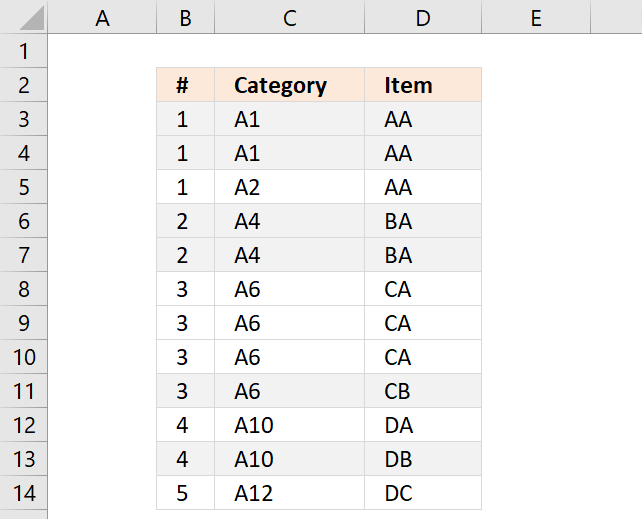Example 2, groups based on values in column B, C and D.

Conditional formatting formula:

=MOD(SUM(1/COUNTIFS(\$B\$2:\$B2, \$B\$2:\$B2, \$C\$2:\$C2, \$C\$2:\$C2, \$D\$2:\$D2, \$D\$2:\$D2)), 2)

Make sure you get the relative and absolute cell references right.

### Explaining CF formula

#### Step 1 - Count equal rows

The COUNTIFS function calculates the number of cells across multiple ranges that equals all given conditions.

The cell references \$B\$2:\$B2 and so on expands automatically when the CF applies the conditional formatting formula to cells below. This makes the formula remember previous rows and is able to highlight correct rows.

COUNTIFS(criteria_range1criteria1, [criteria_range2criteria2]…)

COUNTIFS(\$B\$2:\$B2, \$B\$2:\$B2, \$C\$2:\$C2, \$C\$2:\$C2, \$D\$2:\$D2, \$D\$2:\$D2)

becomes

COUNTIFS(1, 1, "A1", "A1", "AA", "AA")

and returns 1.

#### Step 2 - Divide 1 with result

1/COUNTIFS(\$B\$2:\$B2, \$B\$2:\$B2, \$C\$2:\$C2, \$C\$2:\$C2, \$D\$2:\$D2, \$D\$2:\$D2)

becomes

1/1

and returns 1.

#### Step 3 - Sum numbers

The SUM function adds the numbers in a cell range or array and returns a total.

SUM(1/COUNTIFS(\$B\$2:\$B2, \$B\$2:\$B2, \$C\$2:\$C2, \$C\$2:\$C2, \$D\$2:\$D2, \$D\$2:\$D2)

becomes

SUM(1)

and returns 1.

#### Step 4 - Calculate remainder

MOD(SUM(1/COUNTIFS(\$B\$2:\$B2, \$B\$2:\$B2, \$C\$2:\$C2, \$C\$2:\$C2, \$D\$2:\$D2, \$D\$2:\$D2), 2)

becomes

MOD(1,2)

and returns 1.

Back to top

## 6. How to return the fractional part of a number?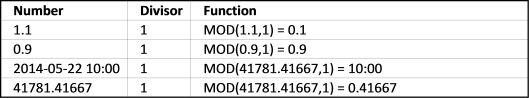If you use 1 as a divisor the MOD function returns the fraction of a number. number - INT(number/divisor)

You can use this to return only hours from a cell containing date and time, see row 3 in the picture above.

Back to top

## 7. How to calculate the integer portion of a division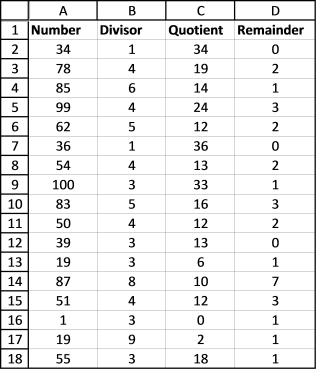The quotient function returns a number without the fractional component.

78 / 4 = 19.5

The quotient is 19. Here are more examples, the quotients are in column C.

QUOTIENT(numerator, denominator) uses the same arguments as the MOD function.

Back to top

## 8. How to remove whole number and return decimal?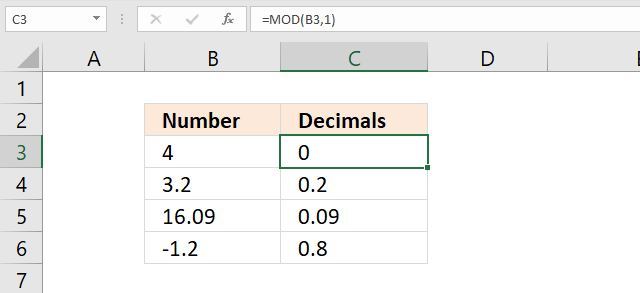The formula in cell C3 removes the whole number and returns only the decimal part.

Formula in cell C3:

=MOD(B3, 1)

The formula above won't work with negative numbers, see cell C6 above.

This formula in cell C3 works with both positive and negative numbers:

=IF(B3<0, -MOD(ABS(B3), 1), MOD(B3, 1))

Back to top

## 9. MOD function won't work with really large numbers

There seems to be a problem with large numbers and Microsoft knows about it:
https://support.microsoft.com/kb/119083

The MOD() function returns the #NUM! error if the following condition is true:
('divisor' * 134217728) is less than or equal to 'number'

Back to top

### Download Excel fileHow-to-use-the-MOD-function.xlsx

## Recommended reading

Extracting Vectors From A Matrix

Back to top